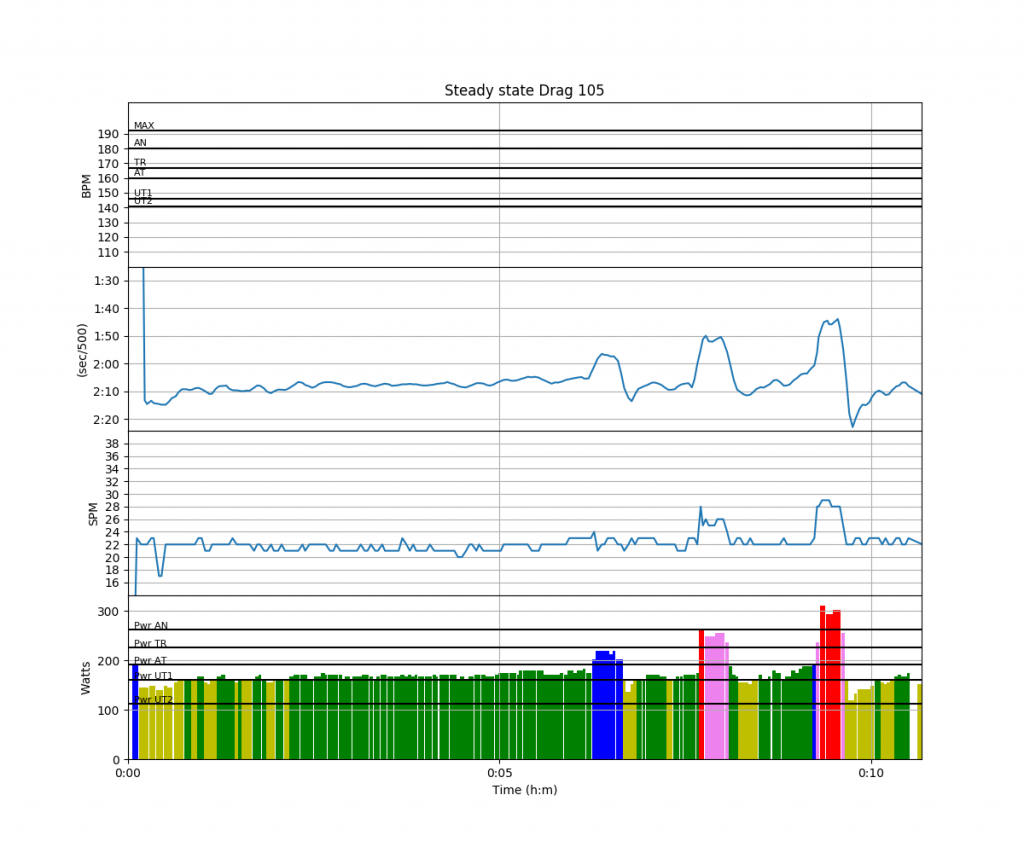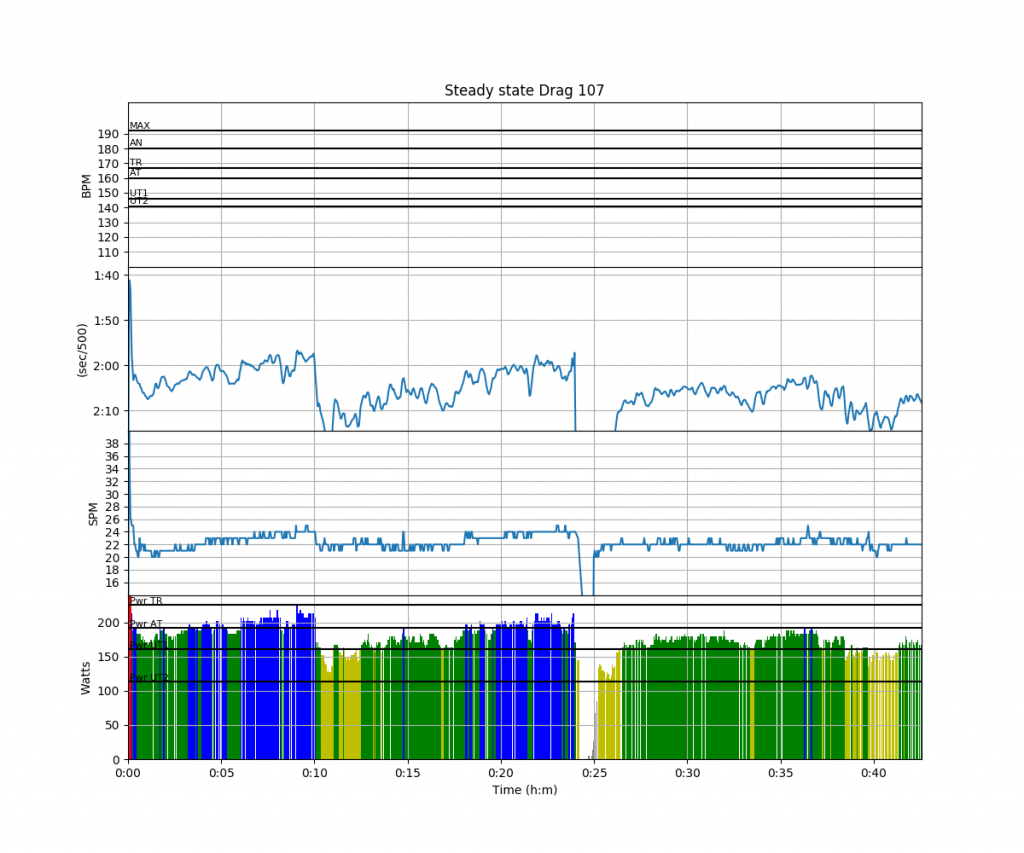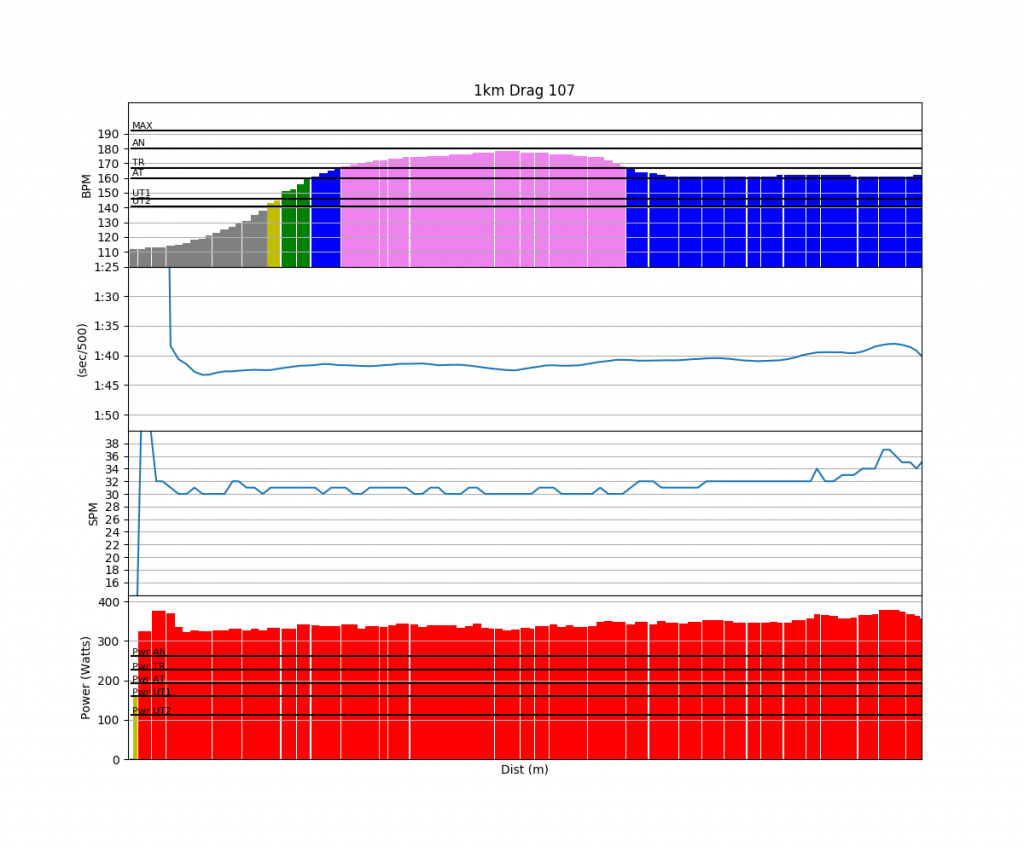# Steady State, Swim, 1k erg and some Math

This is going to be a very brief summary of the past three days of training, plus some math related to a big upgrade planned for rowsandall.com.

Sunday

Steady state at the rowing club. As the others were doing some 10 minute intervals, with increasing intensity during the 10 minute, my steady state was reflecting that, at a milder intensity and a less steep power increase.One hour in total.

Monday

A long day at work and meetings late into the evening. So I went swimming in the morning. I wore the Tickr X heart rate belt, which has an internal memory. Unfortunately, it doesn’t record heart rate well in the pool. The summary said total time 78 minutes (correct), maximum heart rate 161 bpm (could be right), average heart rate 109 bpm (definitely wrong). I think the average is somewhere around 140-145 bpm.

Tuesday

Another long day at work, then a quick dinner, followed by a 1k full out. Twenty five minutes of warming up. Ten minutes of cooling down. My volume is decreasing as a taper for Saturday’s race.Funny heart rate values. I don’t believe them much.

 Workout Summary - media/20180109-1905300o.csv --|Total|-Total-|--Avg--|-Avg-|Avg-|-Avg-|-Max-|-Avg --|Dist-|-Time--|-Pace--|-Pwr-|SPM-|-HR--|-HR--|-DPS --|01000|03:21.4|01:40.7|339.2|31.1|158.2|178.0|09.6 W-|01000|03:21.4|01:40.7|339.4|31.1|159.0|178.0|09.7 R-|00000|00:00.0|00:00.0|000.0|00.0|000.0|178.0|00.0 Workout Details #-|SDist|-Split-|-SPace-|-Pwr-|SPM-|AvgHR|MaxHR|DPS- 00|00094|00:18.0|01:36.0|294.6|27.6|114.3|119.0|11.3 01|00094|00:19.1|01:42.0|329.1|30.8|131.7|145.0|09.5 02|00107|00:21.7|01:41.3|337.3|30.7|161.9|170.0|09.6 03|00097|00:19.6|01:40.9|339.1|30.8|173.3|175.0|09.7 04|00096|00:19.6|01:41.5|334.5|30.2|176.8|178.0|09.8 05|00107|00:21.7|01:41.1|337.6|30.4|175.8|177.0|09.8 06|00095|00:19.1|01:40.4|346.8|31.0|165.1|172.0|09.6 07|00103|00:20.6|01:40.2|347.8|31.7|161.0|161.0|09.4 08|00096|00:19.1|01:39.4|354.2|32.2|161.8|162.0|09.4 09|00111|00:22.1|01:39.4|366.5|34.6|161.3|162.0|08.7 

Well, that was better than expected. I aimed for 330-335W. Not bad for a regular weekday evening, after a long workday.

Finally, Some Math

In case you want to find the highest average power interval of a certain duration in a workout, or perhaps all “fastest” intervals for all interval lengths, you can use the following.

Your workout data is a series of metrics measured at equidistant times$\bf{t} = [ t_1 \, t_2 \dots t_{n-1} \, t_n]$. Similar to the time vector$\bf{t}$ there is a power vector$\bf{P}$. Calculate the vector$\bf{G}$ given by:$G_j = \sum_{i=1}^{j-1} P_i$

We then calculate a matrix$\bf{D}$:$D_{nm} = G_n - G_m$

The average power for an interval starting at$t_n$ and ending at$t_m$ is then given by:$\bar{P}_{nm} = \frac{D_{nm}}{t_m - t_n}$.

If$\bar{P}_i$ represents the$i$th diagonal of$\bf{\bar{P}}$, counting from 0, the 0th diagonal being “the” diagonal$\bar{P}_0 = [\bar{P}_{00} \, \bar{P}_{11} \dots \bar{P}_{n,n} \, \bar{P}_{n+1,n+1}]$,

and$\bar{P}_1 = [\bar{P}_{10} \, \bar{P}_{21} \dots \bar{P}_{n,n-1} \, \bar{P}_{n+1,n}]$

then this vector represents all values of the average power for a time segment of duration$i \Delta t$. Then you simply take maximum value of this vector.

When written like this, the calculation is just a few lines of code, with the help of the numpy library.

So now you know what we do to your rowing data.